# Manipulating Data Bitwise

## Overview

Bitwise operators often are used for extracting and inserting specific ranges of bits. There are two common situations in which you might want to manipulate individual bits within a byte. The first situation involves working with digital communications, such as those used in the digital I/O aspects of DAQ devices. The second situation involves working with data that might contain multiple pieces of information in different bits or sets of bits. For example, if you have an unsigned 32 bit integer (U32), N, which contains value A in the upper 16 bits and value B in the lower 16 bits, you need to use bitwise functions to extract values A and B from N.

## Functions

Several functions are used in bitwise fashion. These functions often are used on entire bytes or larger data types. In this case, we refer to the function as bitwise, which means to operate on each bit within this data type individually. Bits can take on values of either 0 or 1, and all bitwise operators are Boolean in nature. Because the operators are Boolean, LabVIEW uses the same Boolean functions that it uses for Boolean data types to perform bitwise operations.

Since the operators are capable of operating on entire bytes, it is common to see bitwise operators performed on numbers, usually integers. You can convert the numbers to binary representation for a better understanding of the mechanics of the operation.

Note: This document uses C syntax to describe bitwise operators to familiarize the reader with that notation because C is commonly used.

There is one unary operator, the bitwise complement (bitwise NOT) operator. It inverts each bit in the argument. In C syntax, the tilde (~) signifies not. LabVIEW uses the NOT function.

~10000110 = 01111001 => ~134 = 121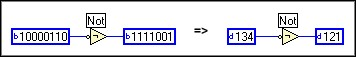The remainder of the bitwise operators, AND ( & ), OR ( | ), and exclusive OR ( ^ ), are binary, and take two arguments. Each binary operator compares each place in both of the arguments bit-to-bit to arrive at a result. For example:

AND
01101100      108
& 10011101    & 157
---------- => -----
00001100       12

OR
01101100      108
| 10011101    | 157
---------- => -----
11111101      253

XOR
01101100      108
^ 10011101    ^ 157
---------- => -----
11110001      241

In LabVIEW, each function uses an equivalent Boolean operator: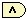,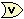, and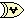.

In addition to the three binary operators mentioned above, there are also two shift operators, left shift (<<) and right shift (>>), which shift the places of the bits. Any bits that need to be added on either end are 0. Any bits that "fall off" either end are ignored.

01101100 << 1 = 11011000   =>   108 << 1 = 216

01101100 >> 1 = 00110110   =>   108 >> 1 = 54

01101100 << 4 = 11000000   =>   108 << 4 = 192

01101100 >> 4 = 00000110   =>   108 >> 4 = 6

The first two examples above appear to multiply and divide by 2, respectively. Right-shifting and left-shifting can be thought of as multiplying by 2^(shift number), as long as none of the bits fall off. However, in the second two examples, bits fall off, and thus the multiplication by 2^(shift number) is no longer valid. Multiplying by that power of two on the same data size also is not valid, for the same reason.

LabVIEW has its own shift operator: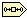. LabVIEW does not differentiate between left- and right-shift functions. The shift operator works like a left shift that can handle negative values. For example, in order to shift right by 4 bits, you must left-shift -4 bits.

## Common Uses for Bitwise Operators

Suppose you communicate with an external digital device that sends you one 8-bit number, N. The upper 4 bits represents quantity A, and the lower 4 bits represent quantity B, both of which you need to extract. Bitwise operators allow for the following:

B = N & 00001111
A = (N & 11110000) >> 4

If you need to reverse the operation and write back to the instrument your values of A and B:

N = B | (A << 4)

In LabVIEW, the Split (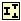) and Join (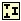) functions perform this exact operation.

Note: LabVIEW cannot split data types smaller than 16 bits, nor can LabVIEW concatenate data types larger than 8 bits.

Another common use for bitwise operators is in what are known as bitfields. Bitfields are normal integer values where each bit specifies some sort of property or flag. If a bitfield were to be used to describe the properties of a LabVIEW control, for instance, bit 0 could describe the visibility state (visible or invisible), bit 1, whether the label was visible, and bit 2, whether the radix was visible, and so on.

Given this, to set a particular bit, bit B in the field N, you would use the following operation:

result = (1 << B) | N

To clear the bit:

result = (~(1 << B)) & N